# Estimating Normals for a Point Cloud

Point clouds aqcuired from 3D sensors often do not come equipped with surface normals. Sensors can, however, always provide a direction vector pointing from a scanned point to origin of the scanner. Point-Cloud-Utils can estimate normals for 3D point clouds, and orient these normals when the user provides sensor direction vectors. The method fits a plane in the neigbhorhood of each point using principle component analysis, and assigns the fitted plane normal to the point. If sensor directions are provided, the normal is flipped to have the same orientation as the sensor direction.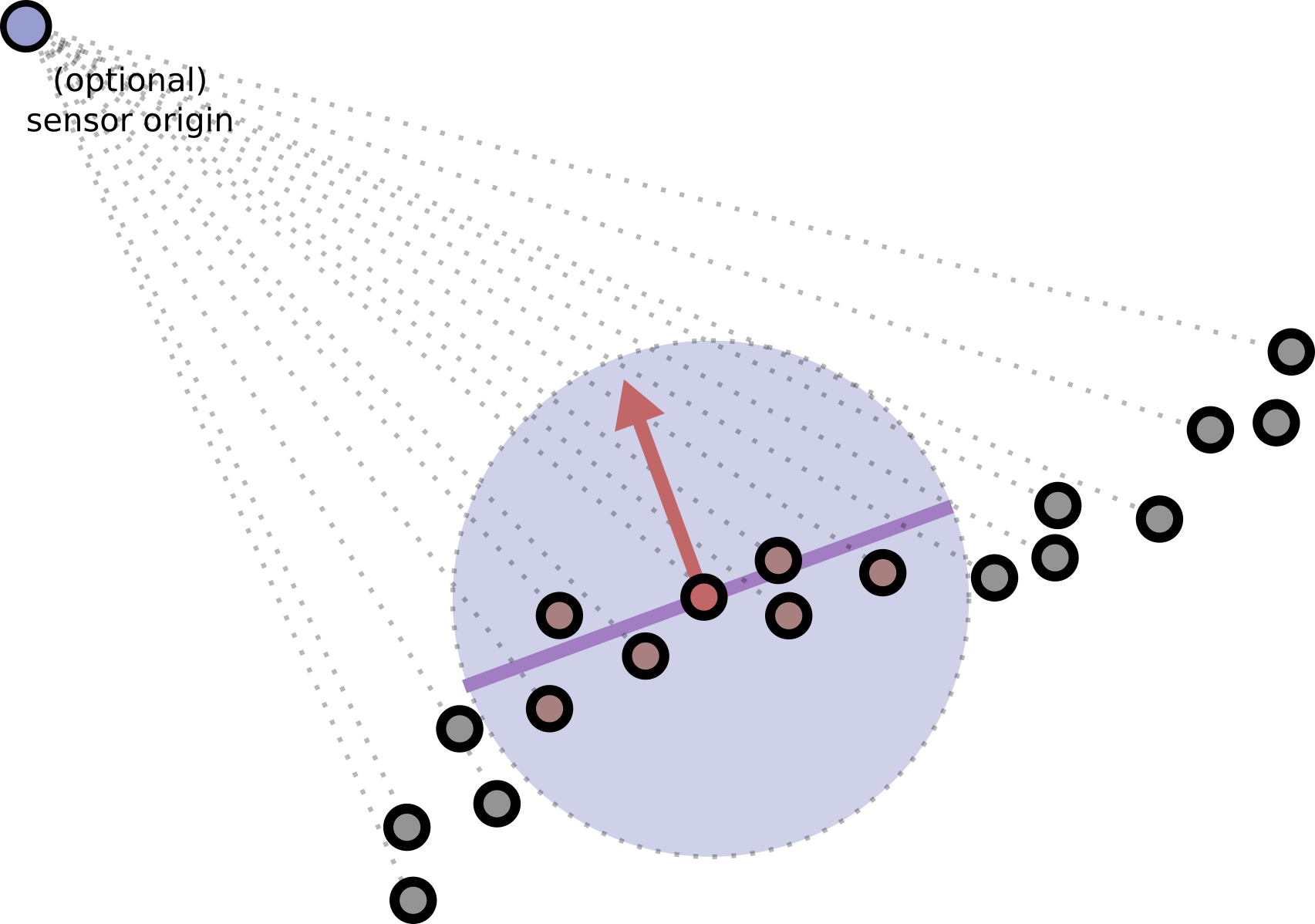Sensor directions are optional

You don't have to pass in sensor directions but then the normals will not be consistently oriented. You should usually be able to get sensor directions from point cloud scans.

## Estimating Normals using k-Nearest-Neighbors

The following code uses the k-nearest neighbors to a point to contruct a local neighborhood for fitting a plane.

import point_cloud_utils as pcu

# sensor_dirs are stored in the normal channel and are encoded as unit
# vectors pointing from the point to the scanner

# Optionally delete point whose normal is at an oblique (greather than 85 degree) angle with the sensor direction

# Size of the neighborhood used for each point
num_nbrs = 32

# n are the fitted normals
# n_idx are used to delete points which were filterd (ignore this if you don't pass in drop_angle)
_, n = pcu.estimate_normals_knn(pts, num_nbrs, view_dirs=sensor_dirs)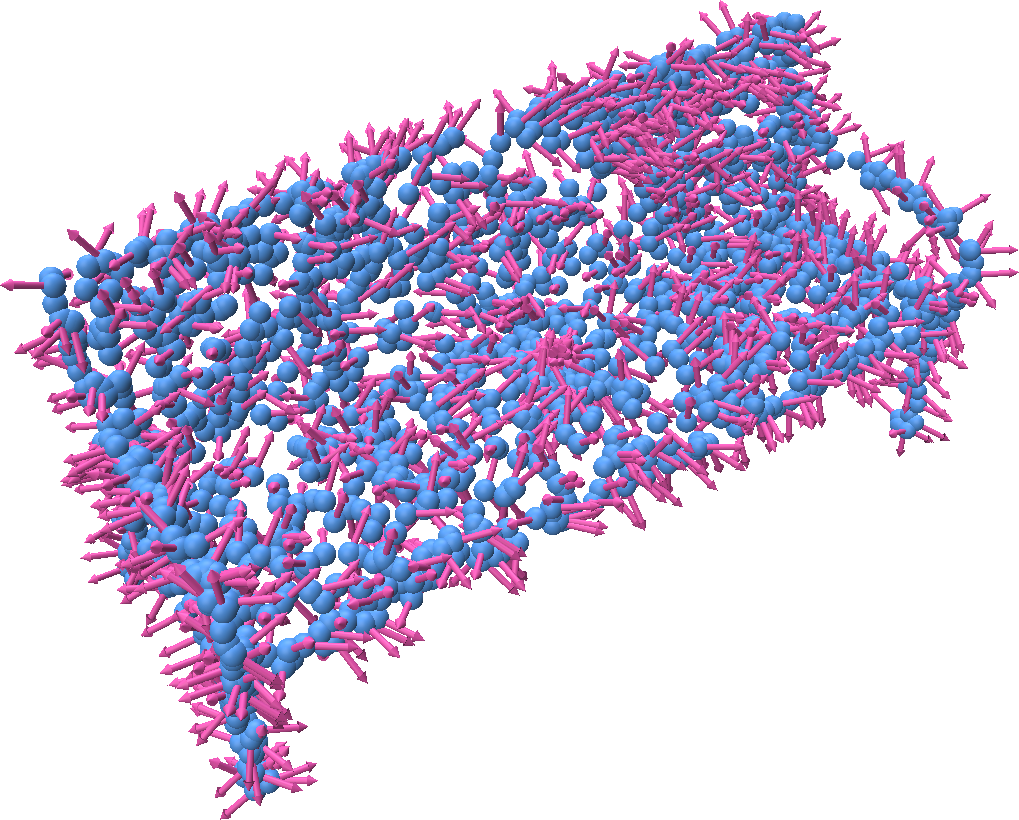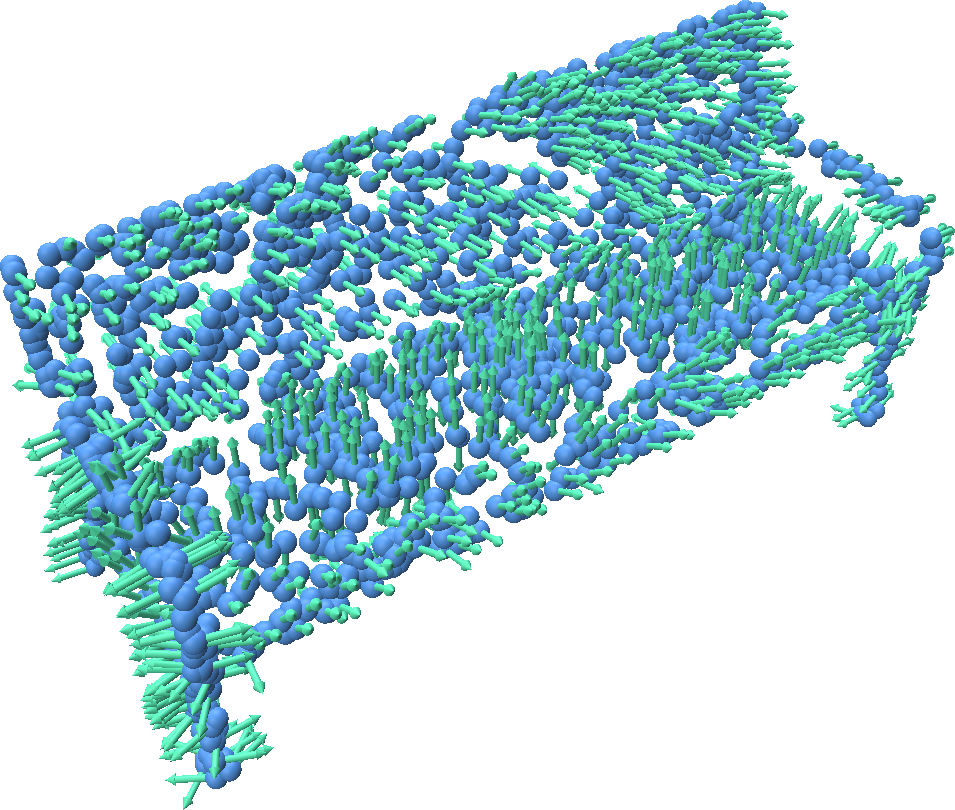### Filterting out points with oblique angles to the sensor

You can optionally filter out points whose predicted normal angle is close to 90 degrees to the sensor direction. This can prevent certain types of noise when reconstructing a surface from oriented points.

import point_cloud_utils as pcu

# sensor_dirs are stored in the normal channel and are encoded as unit
# vectors pointing from the point to the scanner

# Optionally delete point whose normal is at an oblique (greather than 85 degree) angle with the sensor direction

# Size of the neighborhood used for each point
num_nbrs = 32

# n are the fitted normals
# n_idx are used to delete points which were filterd (ignore this if you don't pass in drop_angle)
n_idx, n = pcu.estimate_normals_knn(pts, num_nbrs, view_dirs=sensor_dirs, drop_angle_threshold=drop_angle)

# Only include points which were not dropped
pts_n = pts[n_idx]


## Estimating Normals using a Radius

The following code uses neighbors within a ball around a point to contruct a local neighborhood for fitting a plane.

import point_cloud_utils as pcu

# sensor_dirs are stored in the normal channel and are encoded as unit
# vectors pointing from the point to the scanner

# Optionally delete point whose normal is at an oblique (greather than 85 degree) angle with the sensor direction

# Size of the neighborhood used for each point

# n are the fitted normals
_, n = pcu.estimate_normals_knn(pts, ball_radius, view_dirs=sensor_dirs)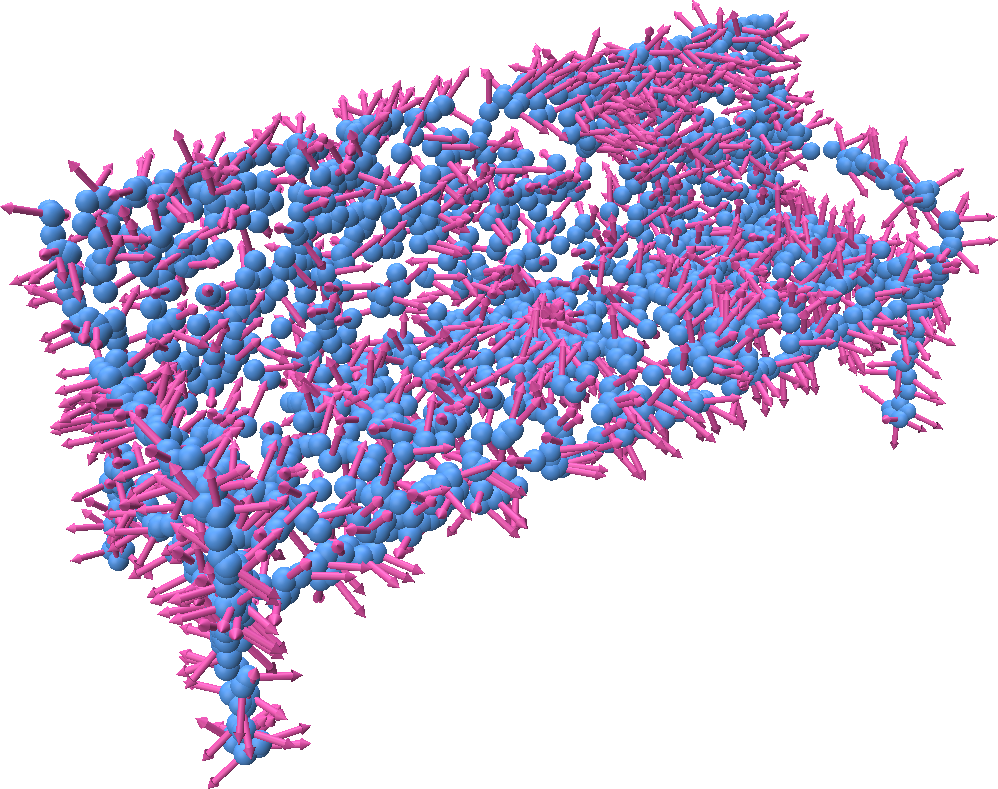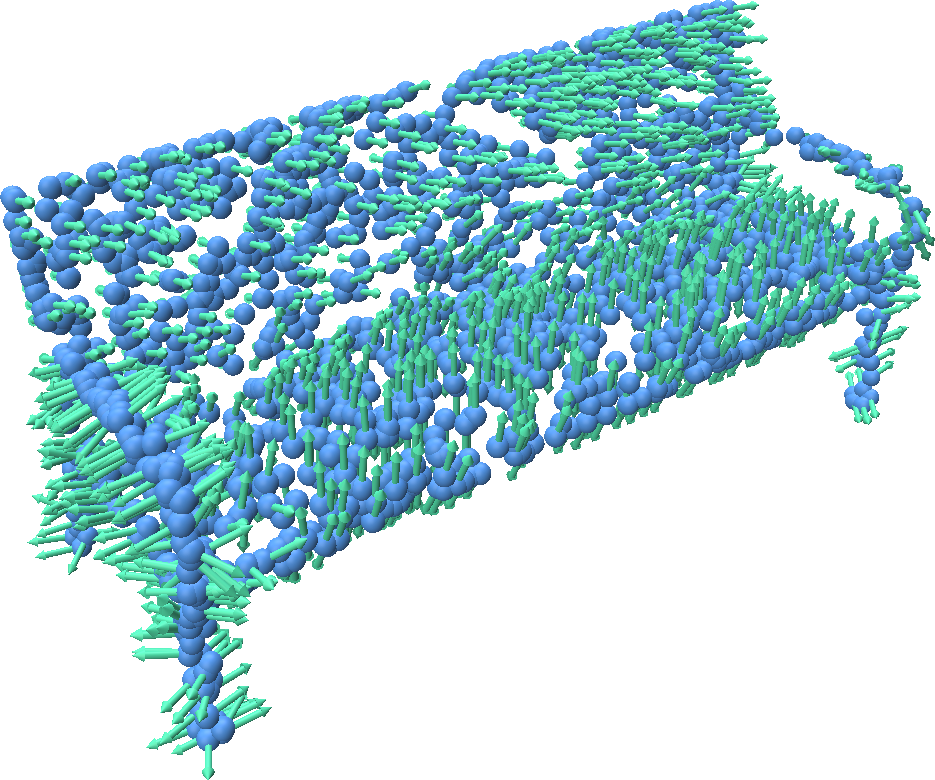### Filterting out points with oblique angles to the sensor

You can optionally filter out points whose predicted normal angle is close to 90 degrees to the sensor direction. This can prevent certain types of noise when reconstructing a surface from oriented points.

import point_cloud_utils as pcu

# sensor_dirs are stored in the normal channel and are encoded as unit
# vectors pointing from the point to the scanner

# Optionally delete point whose normal is at an oblique (greather than 85 degree) angle with the sensor direction